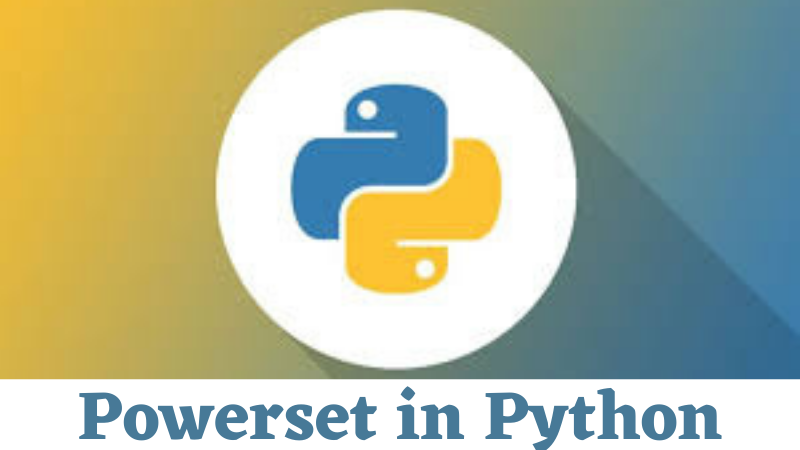/  Technology   /  Powerset in Python## Powerset in Python

A powerset is the set of all the possible subsets of a given set S. A subset is a set that includes no(or)few(or)all the elements of the original set S. It includes both the given set S and an empty set {}.

Given set: S = {1}

Powerset: P = {{},{1}}

Given set: S = {1, 2}

Powerset: P = {{},{1},{2},{1,2}}

Given set: S = {1, 2, 3}

Powerset: P = {{},{1},{2},{3},{1,2},{1,3},{2,3},{1,2,3}}

Python is enabled with itertools.combinations(iterable, n) function which returns subsequences of elements from the input iterable length n. This function can be used to print all the subsets of a set of a specified size. Let’s discuss two ways of doing this.

We pass the set as iterable and the size as arguments in the itertools.combinations() to directly obtain the combination list.

# code to print powerset of given size of a set

```>>import itertools
>>def power_set(s, n):
return list(itertools.combinations(s, n))
>>s = {1, 2, 3}
>>n = 2
print(power_set(s, n))```

Output:

`>>[(1, 2), (1, 3), (2, 3)]`

Here’s another way to print a power set. We use a for loop in itertools.combinations() function and append the list with the combination sets.

#code to print powerset of given size of a set

```>>import itertools
>>def power_set(s, n):
return [set(i) for i in itertools.combinations(s, n)]
>>s = {1, 2, 3, 4}
>>n = 3
>>print(power_set(s, n))```

Output:

`[{1, 2, 3}, {1, 2, 4}, {1, 3, 4}, {2, 3, 4}]`

Reference:

Powerset in Python